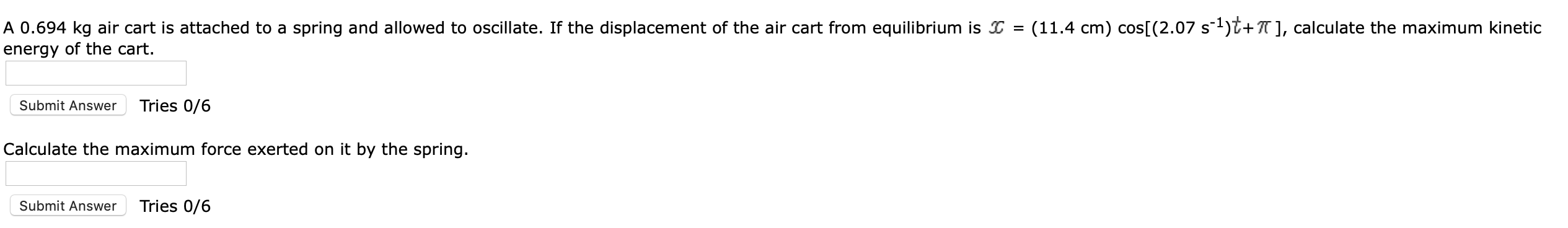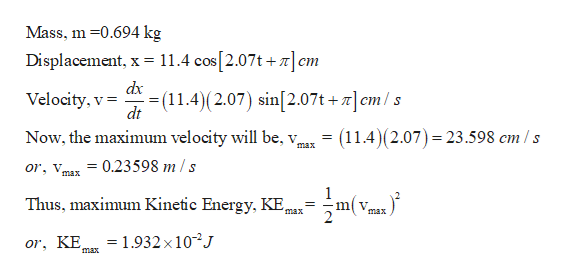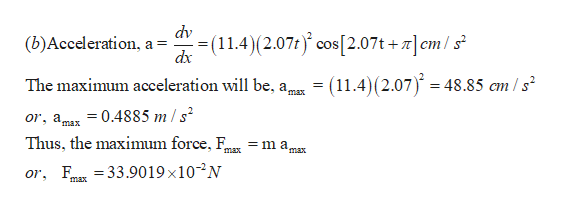# A 0.694 kg air cart is attached to a spring and allowed to oscillate. If the displacement of the air cart from equilibrium isenergy of the cart(11.4 cm) cos[ (2.07 s1)^+], calculate the maximum kinetic=Tries 0/6Submit AnswerCalculate the maximum force exerted on it by the spring.Tries 0/6Submit Answer

Question
1 viewshelp_outlineImage TranscriptioncloseA 0.694 kg air cart is attached to a spring and allowed to oscillate. If the displacement of the air cart from equilibrium is energy of the cart (11.4 cm) cos[ (2.07 s1)^+], calculate the maximum kinetic = Tries 0/6 Submit Answer Calculate the maximum force exerted on it by the spring. Tries 0/6 Submit Answer fullscreen
check_circle

Step 1

(a)help_outlineImage TranscriptioncloseMass, m 0.694 kg 11.4 cos[2.07t+]cm Displacement, x = dx (11.4) (2.07) sin[2.07t +]em /s Velocity, v dt = (11.4)(2.07) = 23.598 cm /s Now, the maximum velocity will be, v, max 0.23598 m/s Or v max Thus, maximum Kinetic Energy, KE, m v max max = 1.932 x 102J or, KE max fullscreen
Step 2

(b)

...help_outlineImage Transcriptionclosedv (11.4) (2.07t cos [2.07t +cml s (b)Acceleration, a = dx = (11.4)(2.07) = 48.85 am /s The maximum acceleration will be, a, max 0.4885 m / s2 or, a max Thus, the maximum force, F max 33.90 19 x 1 0-2N or, max fullscreen

### Want to see the full answer?

See Solution

#### Want to see this answer and more?

Solutions are written by subject experts who are available 24/7. Questions are typically answered within 1 hour.*

See Solution
*Response times may vary by subject and question.
Tagged in

### Other